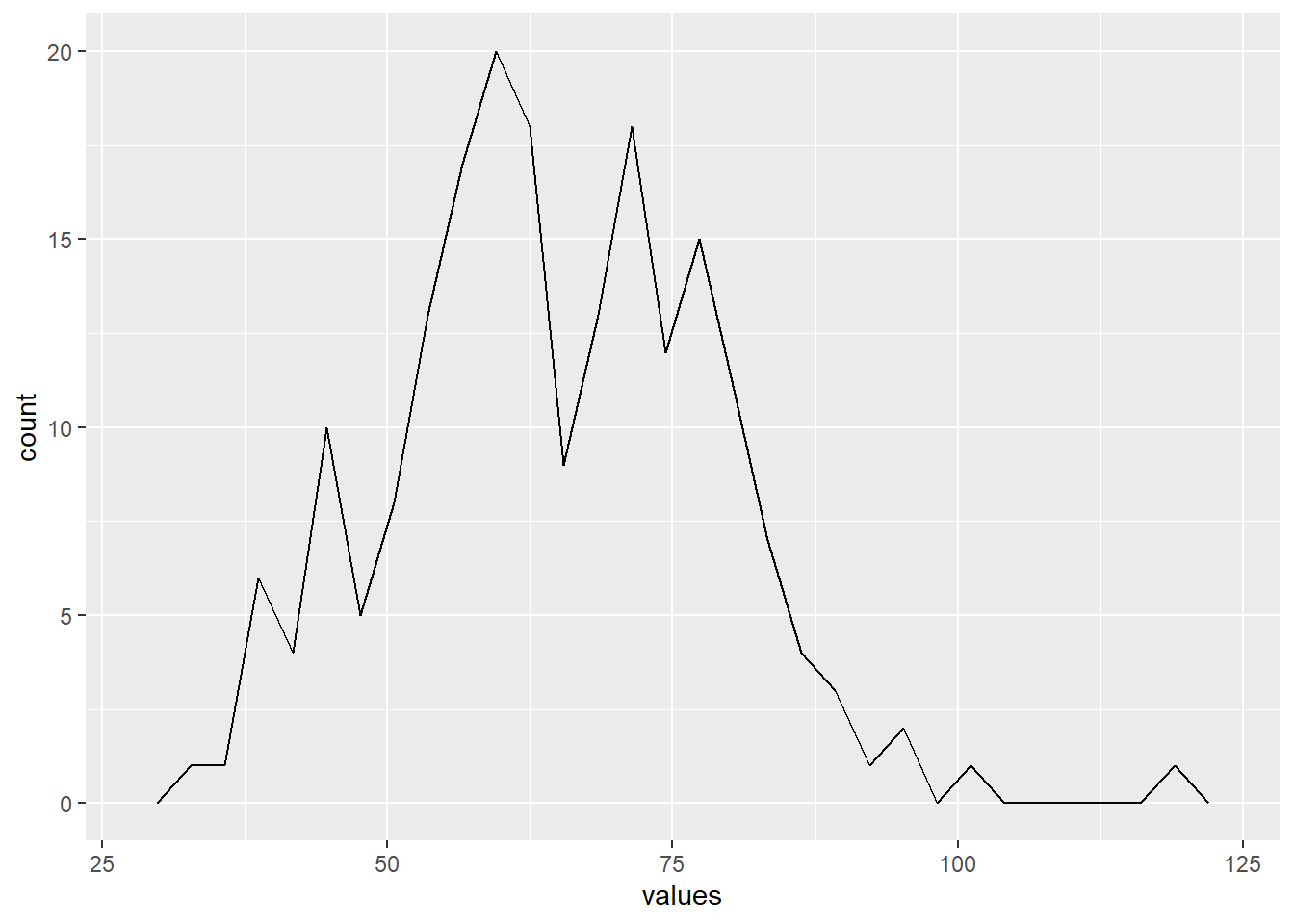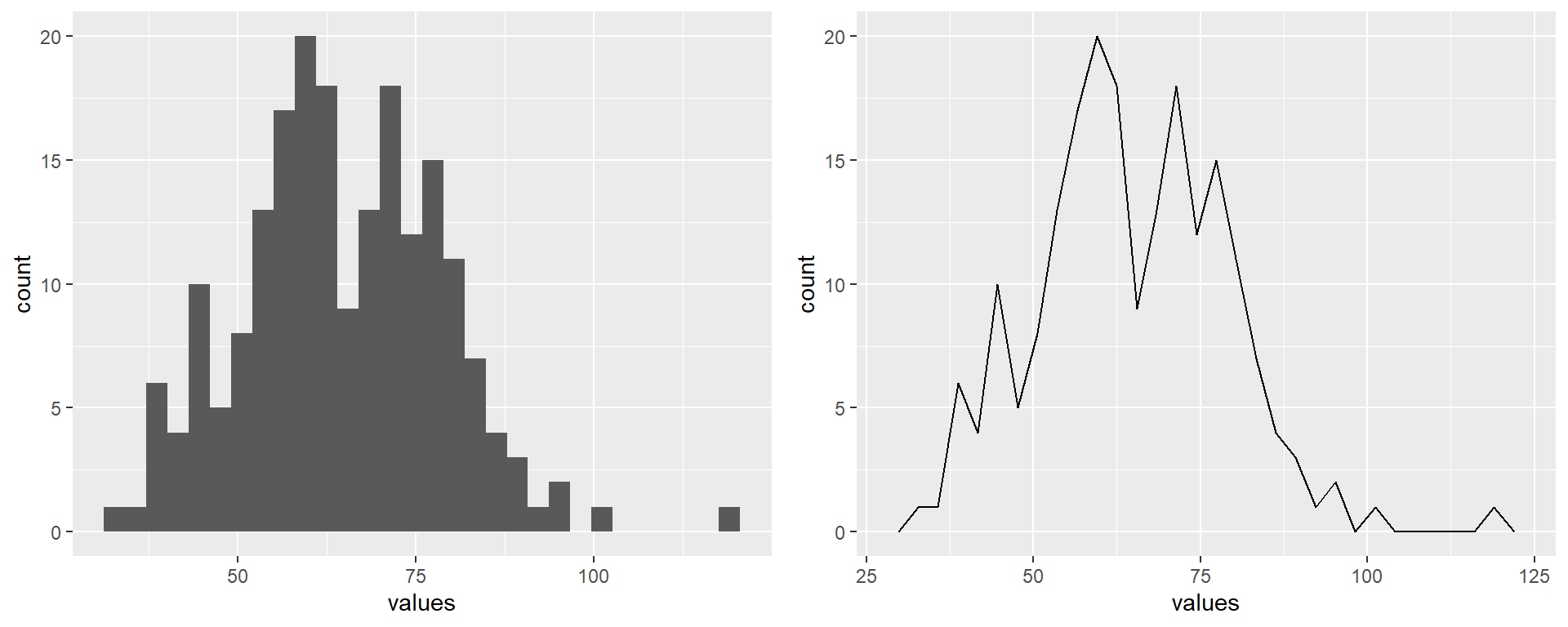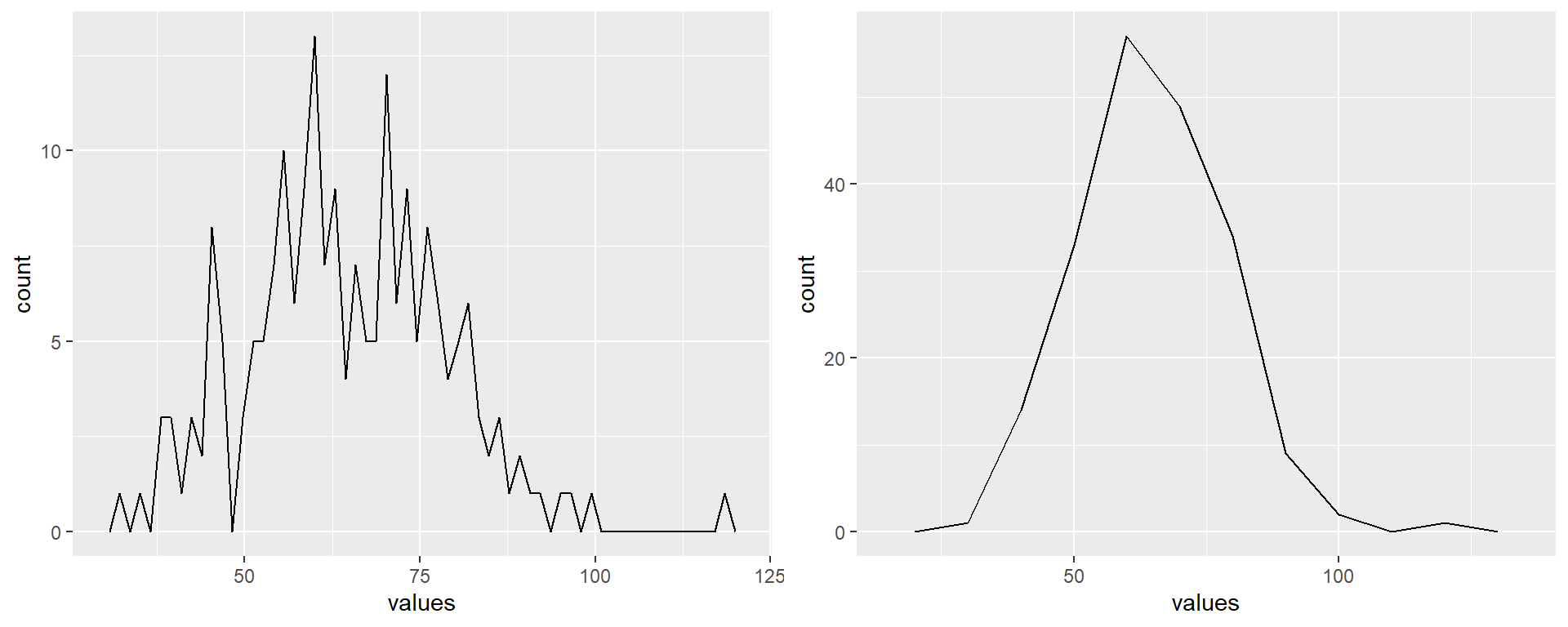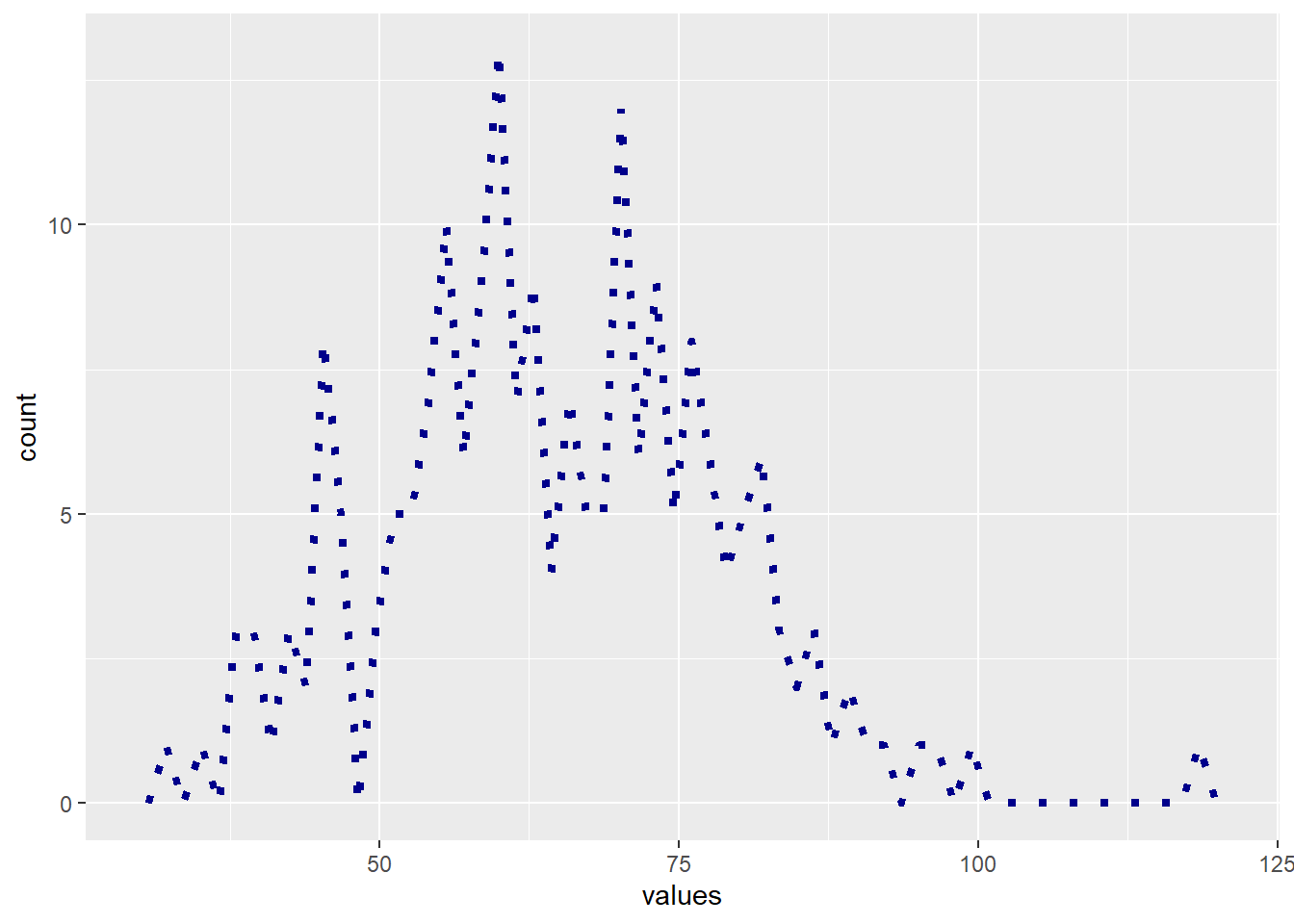A frequency polygon and a histogram are very much alike. Both help visualize the distribution of a data series, the former using bars to represent counts and the latter using lines.

Let’s use ggplot() to draw the frequency polygon for a data set generated by rnorm() (read more here about rnorm()). Here are the variables and dataframe:

# ID
ID <- 1:200
# sample data
values <- rnorm(200, mean=65, sd=15)
# dataframe
df <- data.frame(ID, values)

We first map the data from the variable values by typing ggplot(df, aes(values)) and then use geom_freqpoly() to draw the plot:

ggplot(df, aes(values)) +
geom_freqpoly()
## stat_bin() using bins = 30. Pick better value with binwidth.To realize how similar a frequency polygon and a histogram are, we can put them next to each other:

ggplot(df, aes(values)) +            # histogram
geom_histogram(bins = 30)
ggplot(df, aes(values)) +            # frequency polygon
geom_freqpoly(bins = 30)The shapes of these two plots are not strictly identical but they clearly show the same pattern of distribution.

As for any histogram, we can modify the binwidth or the number of bins using binwidth= or bins= in geom_freqpoly(). Here are two examples:

ggplot(df, aes(values)) +
geom_freqpoly(bins = 60)             # left plot, changing bins
ggplot(df, aes(values)) +
geom_freqpoly(binwidth = 10)         # right plot, changing binwidthAs usual, you may change the look of the line with size=, color= and linetype=:

ggplot(df, aes(values)) +
geom_freqpoly(bins = 60, size = 1.5, color = "darkblue", linetype = "dotted")### Adding plot title, axis titles, ticks, labels and other essential elements

In this section, you will learn how to set/modify all the necessary elements that make a plot complete and comprehensible. Such elements are: